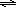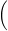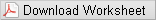# Lab 7 - Buffers

## Purpose

To prepare buffers and measure the pH of each, and to prepare a buffer at a specific pH.

## Goals

• 1
To learn to prepare buffers by both the direct and indirect methods.
• 2
To learn to identify solutions that are buffers.
• 3
To understand the how a buffer resists changes in pH upon addition of acid or base solutions.

## Introduction

In dilute aqueous solutions, weak acids are slightly dissociated. They produce a small concentration of hydronium ion (H3O+) and an equal concentration of the conjugate base of the acid. Such dissociation reactions are equilibria, and equilibrium mathematics can be used to calculate concentrations of the species present in solution. Consider formic acid (CH2O2); its dissociation constant (Ka) is 1.7 x 10-4. Incidentally, formic acid is what red ants inject when they bite. The concentration of H3O+ present in a 0.010 M solution of formic acid can be calculated from the equilibrium expression and a reaction table.
( 1 )
 HCOOH(aq) + H2O(l)H3O+(aq) + HCOO-(aq) initial 0.010 0 0 Delta; -x +x +x equilibrium 0.010 - x x x

( 2 )
Ka = 1.7 x 10−4 =
 [H3O+ ][HCOO− ] [HCOOH]
=
 x2 0.010 − x

Solving for x, one finds that the solution is 0.0012 M in H3O+ and HCOO-.* Expressing [H3O+] as pH
( 3 )
pH = −log[H3O+ ] = log(0.0012) = 2.92
* For this calculation, the quadratic formula was used. If one makes the simplifying assumption that x is small relative to [HCOOH], the calculated value of [H3O+] is 0.0013 M. Although a weak acid dissociates only slightly in water, the soluble salt of a weak acid (for example, sodium formate) is a strong electrolyte and dissociates completely.
( 4 )
HCOONa(s) + H2O(l) → Na+(aq) + HCOO(aq
( 5 )
pH = pKa + log[base] [acid]or pH = pKa + logmoles of base moles of acidThe derivation of this equation follows from the general dissociation equilibrium expression for a weak acid, and includes the assumption that [H3O+] is small relative to [HA]:
( 6 )
HA + H2OH3O+ + A-

( 7 )
Ka =
 [H3O+ ][A− ] [HA]

Solving for [H3O+] gives:
( 8 )
[H3O+] =
 Ka x [HA] [A− ]

Taking the negative logarithm of both sides puts the equation in terms of pH:
( 9 )
pH = − log[H3O+ ] = − log Ka − log([HA]/[A])
By definition, pKa = - log Ka and - log ([HA]/[A- ]) = log ([A- ]/[ HA]). Substituting these terms into equation 9:
( 10 )
pH = pKa + log([A ]/[HA])
Equation 5 shows that pH can be found using either concentrations of acid and base, or the number of moles of each. This follows from the fact that the volume term is the same for the acid and its conjugate base, and cancels in the calculation. The Henderson-Hasselbalch equation shows that the pH of a buffer is close to the pKa of the weak acid from which it is made. The exact pH is dependent on the ratio [A- ]/[HA]. If [HA] = [A- ], log ([A- ]/[HA]) = 0 and the pH of the buffer will be exactly the pKa of the acid. If one wishes to make a buffer of a specific pH, one selects an acid with a pKa near that value and adjusts the ratio of [A- ]/[HA] to obtain the desired buffer. Suppose we want a pH 4.00 formate buffer. The pKa of formic acid is -log (1.7 x 10-4) = 3.77.
( 11 )
 pH = pKa + log([A− ]/[HA]) 4.00 = 3.77 + log([A− ]/[HA]) [A− ]/[HA] = 1.70

A ratio of 1.70 moles of formate anion per mole of formic acid should produce a buffer of the desired pH. Buffer capacity can be controlled by the concentrations of each. A buffer prepared with 0.17 mole of formate and 0.1 mole of formic acid per liter would have ten times the capacity of a buffer containing 0.017 mole of formate and 0.010 mole of formic acid, but the initial pH of both should be the same. In Part A of this experiment, you will prepare various acetate buffer solutions by the direct method and measure the pH of each solution. In the direct method, the conjugate acid and base are added together in solution to get the desired base to acid ratio. For example, acetic acid and sodium acetate will be combined in solution. In Part B, you will prepare acetate buffers by the indirect method and measure the pH of each solution. In the indirect method, strong base is added to the weak acid OR strong acid is added to the conjugate weak base. For example, acetic acid will be mixed with sodium hydroxide solution. Upon mixing, the weak acid and hydroxide ion react to produce acetate ions and water. As long as hydroxide ion is the limiting reagent, and some acetic acid remains, the solution contains both species of the conjugate pair (acetic acid and acetate ions), and a buffer exists. In Part C of the experiment, you will be given a target pH and you will need to prepare a phosphate buffer with the given pH. Phosphoric acid is a polyprotic acid and there are several buffer regions. You will use Na2HPO4 · 7 H2O and another phosphate salt, either NaH2PO4 · H2O or Na3PO4 · 12 H2O. The Henderson-Hasselbalch equation suggests that even at a 10:1 acid:base ratio (or a 1:10 ratio) the pH of the resulting solution will only differ from the pKa by one pH unit. Therefore, you need to select a conjugate pair whose pKa is close to your target pH. The acid/base table shows that the H2PO4-/HPO42- conjugate pair has a pKa of about 7.2, so it should be a good system to use for buffers in the pH range of about 6.5 to 8.0. The HPO42-/PO43- conjugate pair has a pKa of about 12.3, so it should be a good system to use for buffers in the pH range of about 11.5 to 13.0.

## Equipment

• 2
30 mL beakers
• 2
• 1
10.0 mL volumetric pipet
• 1
pipet bulb
• 1
• 1
• 1
50 mL beaker
• 1
100 mL beaker
• 1
glass stir rod
• 1
MicroLab Interface
• 1
MicroLab pH Measurement Instruction Sheet
• 1
pH electrode in pH 7.00 buffer
• 1
ring stand
• 1
clamp
• 1
250 mL beaker for electrode rinsings
• 1
deionized water squirt bottle
• 1
box Kimwipes

## Reagents

• ~10 mL
6.0 M acetic acid (HC2H3O2)
• <10 g
solid sodium acetate trihydrate (NaC2H3O2 · 3 H2O)
• ~25 mL
1.0 M NaOH
• 3 g
solid Na2HPO4 · 7 H2O
• TBD
solid NaH2PO4 · H2O or solid Na3PO4 · 12 H2O
• ~15 mL
pH 4.00 buffer
• ~15 mL
pH 7.00 buffer
• ~15 mL
pH 10.00 buffer
• 1.0 M NaOH in a dropper bottle for Part C
• 1.0 M HCl in a dropper bottle for Part C

## Safety

Acetic acid, HCl and NaOH are corrosive. They can attack the skin and cause permanent damage to the eyes. If one of these solutions splashes into your eyes, use the eyewash immediately. Hold your eyes open and flush with water. If contact with skin or clothing occurs, flush the affected area with water. Have your lab partner notify your instructor about the spill.

## Waste Disposal

All solutions can be flushed down the sink with water.

## Prior to Class

Please read the following section of Lab Safety and Practices: Please read the following section of Lab Equipment: Please review the following videos: Please complete WebAssign prelab assignment. Check your WebAssign Account for due dates. Students who do not complete the WebAssign prelab are required to bring and hand in the prelab worksheet.## Lab Procedure

Please print the worksheet for this lab. You will need this sheet to record your data.In this experiment, you will be using pH electrodes connected to the MicroLab Interface. pH electrodes have a thin glass bulb at the tip. They break easily and are costly to replace. Be careful not to shove the electrode into the bottom of a beaker or drop the electrode. There is a protective guard around the tip, which should remain in place at all times. The guard will not protect against careless treatment. Please use extreme care when using this equipment. Best results in using the electrodes are obtained if:
• Electrodes are kept in standard pH 7 buffer solution when not in use.
• Immediately prior to use, the electrodes are rinsed with deionized water and gently blotted with a tissue, then placed in the test solution.
• The electrodes are rinsed and blotted again after the measurement and returned to the pH 7 buffer solution.

### Part A: Acetate Buffer by the Direct Method

1
Open the MicroLab program.
2
Make sure the pH electrode is connected to the interface.
3
Calibrate the pH electrode using the MicroLab instructions provided in the lab. The calibration standards for the pH electrode will be a pH = 4.00 (red) buffer solution, a pH = 7.00 (yellow) buffer solution, and a pH = 10.00 (blue) buffer solution. Use about 15 mL of each in 30 mL beakers.
4
After the calibration is complete, configure the MicroLab program to collect data as described in the instructions provided in the lab.
5
Using the 10.0 mL volumetric pipet and a 100.0 mL volumetric flask, prepare 100.0 mL of 0.60 M HC2H3O2 by diluting the 6.0 M stock solution provided. (Hint: Remember your prelab exercise!) Be sure to condition your pipet before using it.
Question 1: Show your calculation for preparing the 0.60 M HC2H3O2 solution from your prelab assignment.
6
Using the other 100.0 mL volumetric flask, prepare 100.0 mL of 0.60 M sodium acetate solution by dissolving solid sodium acetate trihydrate (NaC2H3O2 · 3 H2O) in water and diluting to a total volume of 100.0 mL. (Hint: Remember your prelab exercise!)
Question 2: Show the calculation of how many grams of NaC2H3O2 · 3 H2O are needed from your prelab assignment.
7
Use a graduated cylinder to measure 30 mL of the 0.60 M acetic acid solution you prepared into a 50 mL beaker. Measure the pH of this solution and record it in Data Table A as solution 1A. Make sure the electrode bulb is fully immersed before measuring.
Table A: pH Data for Acetate Buffers (Direct Method)
8
Add 10 mL of your sodium acetate solution to the beaker containing acetic acid and stir with a clean stirring rod. Measure the pH of the solution and record it in Data Table A as solution 2A. After the measurement is complete, the solution may be discarded.
9
Use a graduated cylinder to measure 30 mL of the 0.60 M sodium acetate solution you prepared into a 50 mL beaker. Measure the pH of the solution and record it in Data Table A as solution 3A.
10
Add 10 mL of your acetic acid solution to the beaker containing sodium acetate and stir with a clean stirring rod. Measure the pH of the solution and record it in Data Table A as solution 4A. After the measurement is complete, the solution may be discarded.
11
Make solution 5A by mixing 20 mL of acetic acid solution with 20 mL of sodium acetate, stirring well. Measure its pH and record it in Data Table A as solution 5A.
Question 3: Explain the order of pH for the five solutions. Consider the relative amounts of acid and base in each.

### Part B: Acetate Buffer by the Indirect Method

1
Place 30 mL of your 0.60 M acetic acid in a clean 100 mL beaker. Measure the pH of the solution and record it in Data Table B as solution 1B.
Table B: pH Data for Acetate Buffers (Indirect Method)
2
Determine whether or not this solution is a buffer solution, and enter your decision in Data Table B.
Question 4: How many mmol of acetic acid are present in your sample 1B? Show your work.
3
Add 4 mL of 1.0 M NaOH and mix the solution thoroughly. Measure the pH of the solution and record it in Data Table B as solution 2B.
Question 5: How many total mmol of NaOH have you added at this point? (Show your setup.) Enter this amount in Data Table B.
Question 6: Is this solution a buffer solution? Explain your reasoning. Fill in Data Table B with your choice.
4
Add in succession another 5 mL, then 6 mL and finally 10 mL of 1.0 M NaOH, mixing thoroughly and recording the pH after each addition.
5
Enter the cumulative number of moles of NaOH added in each step in Data Table B. After the last addition, a total of 25 mL of NaOH should have been added.
6
Decide whether or not each solution is a buffer and enter your decision in Data Table B.

### Part C: Phosphate Buffer by the Direct Method

1
Your lab instructor will assign you a target pH; write it in Data Table C. You need to generate 100 mL of the buffer solution starting with 3.00 g of Na2HPO4 · 7 H2O
2
Weigh out 3 g of Na2HPO4 · 7 H2O and record the exact mass in Data Table C. Transfer it carefully to a 100 mL volumetric flask. Add about 60 mL of deionized water, stopper the flask, and shake it to dissolve the solid. This will take a while.
Question 7: Will you need to use Na3PO4 · 12 H2O or NaH2PO4 · H2O in order to generate the desired pH? Explain your answer. Enter your choice in Data Table C.
Question 8: What mass of the other phosphate compound will you need to add in order to generate the desired pH? Show your work. Enter this amount in Data Table C.
3
Weigh out the calculated amount of the second phosphate compound and carefully add it to your volumetric flask. Stopper the flask and shake until all solid is dissolved. Then add deionized water up to the mark of your flask.
4
Pour some of the buffer solution into a beaker and measure its pH. Record the result in Data Table C.
Table C: Data for Phosphate Buffer
5
If the pH is different than the target pH, adjust it by adding 1.0 M HCl or 1.0 M NaOH dropwise until the desired pH is achieved. In your notebook, describe the action taken to adjust the pH of the buffer, e.g. "added 3 drops of 1.0 M HCl".
6
After your last measurement, stop and close the MicroLab software. Rinse all of your glassware with water, dry it and return it to the set-up area where you found it. Make sure the pH electrode is submerged in the pH 7 buffer solution.
7
Before leaving, enter your results in the in-lab assignment. If all results are scored as correct, log out. If not all results are correct, try to find the error or consult with your lab instructor. When all results are correct, note them and log out of WebAssign. The in-lab assignment must be completed by the end of the lab period. If additional time is required, please consult with your lab instructor.